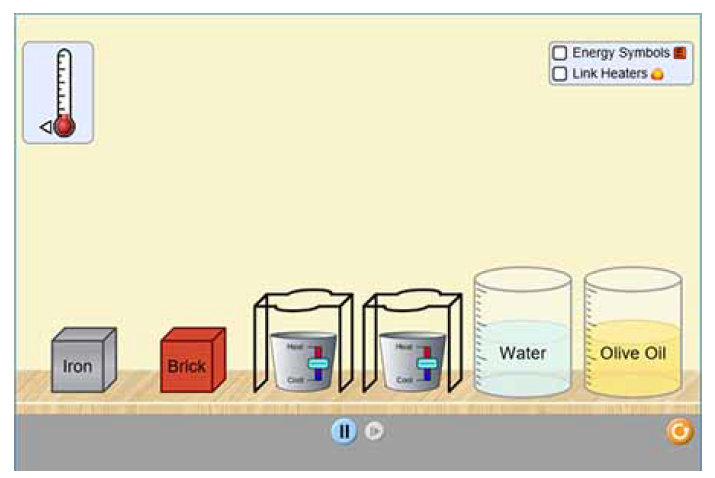# Problem: A 1.5 kg block of iron at 36°C is rapidly heated by a torch such that 13 kJ is transferred to it. What temperature would the block of iron reach (assuming the complete transfer of heat and no loss to the surroundings)? If that same amount of heat (13 kJ ) was quickly transferred to a 850 g pellet of copper at 37°C, what temperature would the copper reach before it begins losing heat to the surroundings? Use the equation for heat capacity and the following heat capacity values:q = mcsΔTcs, Fe ( s ) = 0.450 J/ (g°C)cs, Cu ( s ) = 0.385 J/ (g°C)Express the final temperatures of the iron and copper in °C to two significant figures separated by a comma.Energy can exist in a variety of forms. Energy is never lost, but rather it is converted between forms. When examining a system, the internal energy of that system can change such that it loses or gains energy to or from its surroundings, and the form of energy does not matter. The change in the internal energy of a system isΔE = Efinal − Einitialwhich can also be simply represented byE = q + wwhere q signifies the heat absorbed (or released) by the system, and w signifies the work done on a system.The values for q and w can be negative if the system loses heat or performs work,respectively. The processes of energy transfer can be described as endothermic (where endo indicates energy going into the system) or exothermic (where exo indicates energy leaving the system).Click on the image to explore this simulation, which shows how energy is transferred between objects in either the same form or through conversions. When you click the simulation link, you may be asked whether to run, open, or save the file. Choose to run or open it.When the simulation is opened, you will see two options. Select the image labeled Intro, which will open to see various objects that can be placed on a heating/cooling surface. Checking the “Energy Symbols” box in the upper right allows you to view the internal energy of the objects and the transfer of thermal energy. Clicking the Systems tab of the simulation allows you to view energy conversions by connecting different energy-producing and energy-converting sources. There are twelve different working configurations for these sources.

###### FREE Expert Solution
93% (403 ratings)View Complete Written Solution
###### Problem Details

A 1.5 kg block of iron at 36°C is rapidly heated by a torch such that 13 kJ is transferred to it. What temperature would the block of iron reach (assuming the complete transfer of heat and no loss to the surroundings)? If that same amount of heat (13 kJ ) was quickly transferred to a 850 g pellet of copper at 37°C, what temperature would the copper reach before it begins losing heat to the surroundings? Use the equation for heat capacity and the following heat capacity values:

q = mcsΔT
cs, Fe ( s ) = 0.450 J/ (g°C)
cs, Cu ( s ) = 0.385 J/ (g°C)

Express the final temperatures of the iron and copper in °C to two significant figures separated by a comma.

Energy can exist in a variety of forms. Energy is never lost, but rather it is converted between forms. When examining a system, the internal energy of that system can change such that it loses or gains energy to or from its surroundings, and the form of energy does not matter. The change in the internal energy of a system is
ΔE = Efinal − Einitial

which can also be simply represented by

E = q + w

where q signifies the heat absorbed (or released) by the system, and w signifies the work done on a system.

The values for q and w can be negative if the system loses heat or performs work,
respectively. The processes of energy transfer can be described as endothermic (where endo indicates energy going into the system) or exothermic (where exo indicates energy leaving the system).

Click on the image to explore this simulation, which shows how energy is transferred between objects in either the same form or through conversions. When you click the simulation link, you may be asked whether to run, open, or save the file. Choose to run or open it.When the simulation is opened, you will see two options. Select the image labeled Intro, which will open to see various objects that can be placed on a heating/cooling surface. Checking the “Energy Symbols” box in the upper right allows you to view the internal energy of the objects and the transfer of thermal energy.

Clicking the Systems tab of the simulation allows you to view energy conversions by connecting different energy-producing and energy-converting sources. There are twelve different working configurations for these sources.